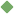calculate 1. [ verb ] (mathematics) make a mathematical calculation or computation Synonyms: compute figure reckon cypher work_out cipher Related terms: reason process estimate multiply subtract add divide resolve factor budget average recalculate interpolate prorate survey capitalize miscalculate extract quantise differentiate integrate mathematics solve count_on bet work_out zero calculation computer calculation calculator calculus calculator To share this definition press "text" (Facebook, Twitter) or "link" (blog, mail) then paste text link 2. [ verb ] judge to be probable Synonyms: figure estimate reckon forecast count_on Related terms: judge allow calculation prognosis 3. [ verb ] predict in advance Synonyms: forecast Related terms: predict prediction forecaster calculation 4. [ verb ] keep an account of Synonyms: account Related terms: charge balance credit debit compound overbalance account calculation calculation accounting accounting 5. [ verb ] have faith or confidence in Synonyms: count depend look reckon bet Examples: "you can count on me to help you any time" "Look to your friends for support" "You can bet on that!" "Depend on your family in times of crisis" Related terms: trust dependence 6. [ verb ] specifically design a product, event, or activity for a certain public Synonyms: aim direct Related terms: intend Similar spelling: calculated   calculate_on   calyculate   calculative   calculator   calculable   calceolate   calculation   calculating   calculus   calycular   callously   caligula   calicular   calculous   calceolaria